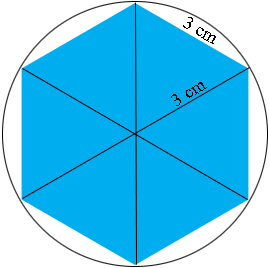SEARCH HOMEMath Central Quandaries & QueriesQuestion from Denise, a teacher: I am being asked to find the volume of a hexagonal prism, base edges are 3cm, and a height of 15cm. The book that I am working from says that the answer is 77.94cm(cubed) but no matter what the equation I use I always get the answer 116.9/117. Please help! Thank you!Hi Denise,

I assume the book means a regular hexagon. First I did a "back of the envelope" calculation to approximate the volume.If the base were a circle of radius 3 cm rather than a hexagon then the area of the base would be $\pi r^2 = \pi \times 3^2.$ but $\pi$ is approximately $3$ so the area of the base is $3 \times 3^2 = 27$ square centimeters.The height is $15$ cm so the volume is approximately $27 \times 15 = 405$ cubic centimeters.

At this point I am not convinced by the book's answer or yours.

In an answer to a previous question Stephen gave an expression for the area of a regular hexagon with $n$ sides and side length $a$ units. The expression is

$\frac{a^2 n}{4\tan\left(\frac{360^o}{2n}\right)}$

For $n = 6$ I get $\tan\left(\frac{360^o}{2n}\right) = \tan(30^o) = \frac{1}{\sqrt3}$ and the area is then

$\frac{3^2 \times 6 \times \sqrt{3}}{4} = 23.38 \mbox{ square centimeters.}$

The volume is then $23.38 \times 15 = 350.74$ cubic centimeters.

I am not sure where we differ but my answer is $3$ times yours.

PennyMath Central is supported by the University of Regina and The Pacific Institute for the Mathematical Sciences.